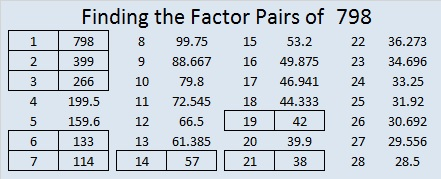# 798 Cupid’s Arrow and Target

Here are two puzzles that go together and yet look out of sync. Sometimes cupid’s arrow reaches its target, and sometimes it doesn’t.Print the puzzles or type the solution on this excel file: 12-factors-795-799

Here’s a little about the number 798:

798 is made from three consecutive numbers (7, 8, and 9), so it is divisible by three. The middle number, 8, is not divisible by three, so 798 is NOT divisible by nine.

798 is a palindrome in two bases:

• 666 BASE 11 because 6(121) + 6(11) + 6(1) = 798
• 383 BASE 15 because 3(225) + 8(15) + 3(1) = 798

798 is also the sum of two consecutive prime numbers: 397 + 401 = 798.

798 can be written as the sum of three squares four different ways:

• 26² + 11² + 1² = 798
• 25² + 13² + 2² =798
• 23² + 13² + 10² = 798
• 22² + 17² + 5² = 798

Here is 798’s factoring information:

• 798 is a composite number.
• Prime factorization: 798 = 2 x 3 x 7 x 19
• The exponents in the prime factorization are 1, 1, 1, and 1. Adding one to each and multiplying we get (1 + 1)(1 + 1)(1 + 1)(1 + 1) = 2 x 2 x 2 x 2 = 16. Therefore 798 has exactly 16 factors.
• Factors of 798: 1, 2, 3, 6, 7, 14, 19, 21, 38, 42, 57, 114, 133, 266, 399, 798
• Factor pairs: 798 = 1 x 798, 2 x 399, 3 x 266, 6 x 133, 7 x 114, 14 x 57, 19 x 42, or 21 x 38
• 798 has no square factors that allow its square root to be simplified. √798 ≈ 28.24889.This site uses Akismet to reduce spam. Learn how your comment data is processed.